Next: Fit results Up: Monte Carlo analysis Previous: MOD yield

## Time-of-flight analysis

In this section, we shall extract two important physics parameters, the rate for the resonant molecular formation and its resonant energies. We show in Fig. 8.19 the results of our Monte Carlo calculations for a 3 T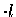DS layer using the nominal input to illustrate the features of our TOF measurements. Plotted are simulated two dimensional scatter-plots of the fusion time and the energy at which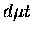formation takes place. The direct events (top) refer to a process in which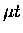formsdirectly, before being scattered by D2 in the DS layer, while the total events (bottom) include also indirect processes where thefirst loses energy in collision with another D2 molecule in the DS layer. The direct process shows a strong correlation between the fusion time and formation energy exhibiting individual resonance structure as shown on the projection on the time axis, whereas for the total events the correlation is obscured by the indirect processes. The role of the indirect processes is more prominent, hence the correlations further weakened, in thick DS layer measurements as shown Fig. 8.20, in which only the total events are plotted (the direct process is nearly independent of layer thickness in these examples). In addition, the thick layer measurements are sensitive to the low energy process as also seen in the US measurement, thus there are relatively large theoretical uncertainties due to solid state effects (notice that a considerable amount of fusion is taking place at very low energies in Fig. 8.20). This is why we focused our measurements and analysis on thin layers. It should be stressed, however, that despite the presence of the indirect process, a significant correlation is preserved between the fusion time and formation energy in the thin layer measurements.

In extracting physical quantities, formation rate and resonance energy, our approach is to perform iterative fits to the data using Monte Carlo calculations with varied input parameters. We varied the formation rate and the resonant energies by scaling

 (114) (115)

where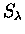is taken as energy-independent, and SE, rate-independent. The fusion probability W was kept at Faifman's value during scaling.

We made full use of our accurate absolute fusion yield determination. In each fit (i.e., fit to each Monte Carlo result for a particular physics scaling input), the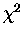was minimized by varying the overall normalization by the Monte Carlo spectrum by a factor . But since our MC yields are already normalized, taking into account factors such as the number of incident muons, the muon stopping fraction, and the Si solid angle, together with experimental corrections due to nitrogen contamination (if present),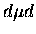proton contribution, the energy cut efficiency, and systematic effect in the background subtraction, all of which have been discussed in great detail in this thesis, we expect in an ideal case. Thus constraining to its uncertainty (which is the relative error in our overall normalization), we define ouras:

 (116)

where hexpi, hMCi are experimental and simulated counts in bin i, with , being the respective uncertainties. Thus the likelihood function is maximized, henceminimized, when the shape and the yield match between experiment and Monte Carlo.Next: Fit results Up: Monte Carlo analysis Previous: MOD yield